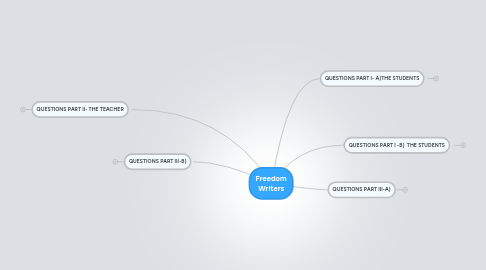# Freedom Writers

Get Started. It's Free
or sign up with your email addressFreedom Writers1.1.1. Answer:

1.2.1. Answer:

1.3.1. Answer:

1.4.1. Answer:

1.5.1. Answer:

## 2. QUESTIONS PART I -B) THE STUDENTS

### 2.1. (6)Think about your own school or out-of-school activities. Are youth respectful of each other’s differences? Are there problems similar to those in Wilson High? If so, what steps can you take to initiate change?

2.1.1. Answer:ahfehfh

2.2.1. Answer:

2.3.1. Answer:

2.4.1. Answer:

2.5.1. Answer:

3.1.1. Answer

3.2.1. Answer

3.3.1. Answer

3.4.1. Answer

3.5.1. Answer

4.1.1. Answer:

4.2.1. Answer:

4.3.1. Answer

4.4.1. Answer

4.5.1. Answer

5.1.1. Answer

5.2.1. Answer

5.3.1. Answer

5.4.1. Answer